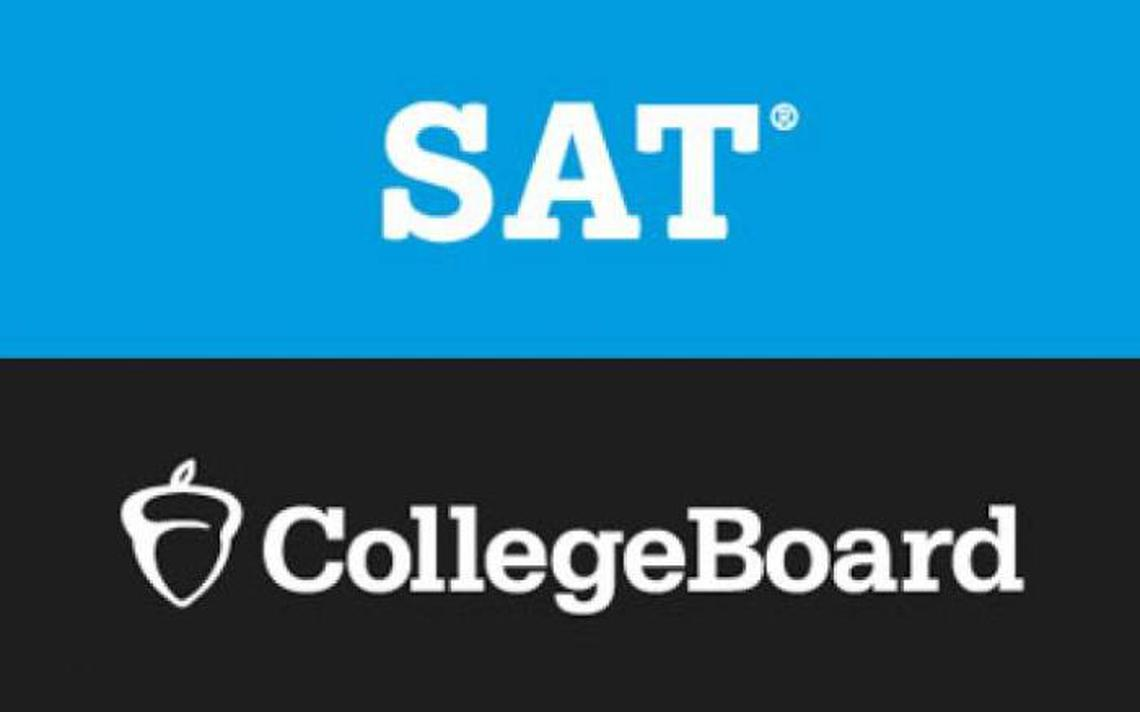SAT is a registered trademark of CollegeBoard and is not affiliated with this website.

### SAT

Get into the college of your dreams!

The SAT, similar to the ACT, is used to assess what you have learned while in school. It has four sections which include Reading, Writing and Language, Math Test - No Calculator and Math Test - Calculator. The scores range from 400 to 1600. Since each high school is different, it is hard to determine if you have two students with a 3.5 which one is more prepared for college. One school might be easier than the other. The SAT is way to test everyone over the same material to see who is more prepared. The SAT is not an IQ test. It tests knowledge someone should had obtained in school. Colleges use this test to help determine who will be admitted and who is eligible for scholarships.

The math section covers four main areas: Heart of Algebra, Problem Solving and Data Analysis, Passport to Advanced Math, and Additional Topics in Math.

Heart of Algebra includes topics linear expressions, linear equations, linear inequalities, linear functions, systems of linear equations and graphs of linear functions.

Problem Solving and Data Analysis includes topics ratios, rates, proportions, percentages, units, unit conversions, linear graphs, quadratic graphs, exponential graphs, linear growth, exponential growth, two way tables, population parameters, mean, mode, median, standard deviation and range.

Passport to Advanced Math includes topics quadratic functions, exponential functions, exponents, polynomial expressions, equations with radicals, solving equations with variable in denominator, rational expressions, nonlinear equations, zeros and factors or polynomials, function notation and literal equations.

Additional Topics in Math includes topics volume formulas, trigonometric functions and pythagorean theorem, complex numbers, degrees and radians, circles, congruency, right triangles, sine, cosine, and complementary angles.

There are some practice test in the Official SAT Study Guide. These tests can also be found on the SAT website for download. Also you can find some old administred tests on the internet.

Looking for tutoring for the SAT Math section? We provide one on one tutoring to improve your SAT Math score.